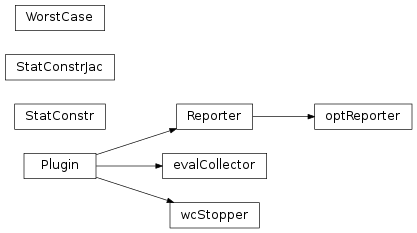# 6.2. `pyopus.design.wc` — Worst case performance computation¶Worst case analysis (PyOPUS subsystem name: WC)

Computes the worst case performance of a circuit. Statistical parameters are assumed to be independent with zero mean and standard deviation equal to 1.

class `pyopus.design.wc.``WorstCase`(heads, analyses, measures, statParamDesc, opParamDesc, fixedParams={}, variables={}, debug=0, beta=3.0, linearWc=True, alternating=True, maxPass=20, wcStepTol=0.01, stepTol=0.01, constrTol=0.01, angleTol=15, stepScaling=4, perturbationScaling=64, screenThreshold=0.01, maximalStopperStep=0.5, evaluatorOptions={}, sensOptions={}, screenOptions={}, opOptimizerOptions={}, optimizerOptions={}, spawnerLevel=1)

See `PerformanceEvaluator` for details on heads, analyses, measures, and variables.

Statistical parameters and operating parameters are given by dictionaries statParamDesc and opParamDesc. These dictionaries have parameter name for key and parameter property dictionary with lo and hi specifying the lower and the upper bound. The nominal value of the operating parameters is given by the `init` member.

Fixed parameters are given by fixedParams - a dictionary with parameter name for key and parameter value for value. Alternatively the value can be a dictionary in which case the `init` member specifies the parameter value.

If fixedParams is a list the members of this list must be dictionaries describing parameters. The set of fixed parameters is obtained by merging the information from these dictionaries.

Setting debug to a value greater than 0 turns on debug messages. The verbosity is proportional to the specified number.

beta is the maximal distance from the origin in the space of statistical parameters. If it is a vector or a list the worst cases corresponding to all values are computed.

If linearWc is set to `True` the initial point for statistical parameters is chosen using linear worst case analysis. Otherwise the initial point is at the origin.

If alternating is setto `True` the worst case is computed by alternating optimizations in the operating and statistical parameter space.

maxPass is the maximum number of algorithm passes (main optimization runs).

wcStepTol specifies the step tolerance for the optimization in the space of operating parameters.

stepTol is the step tolerance for stopping the main optimization algorithm.

constrTol is the constraint violation tolerance for stopping the algorithm.

angleTol is the gradient angle tolerance in degrees for stopping the algorithm.

stepScaling is the divider of the lo-hi range for computing the problem scaling.

perturbationScaling is the divider for the lo-hi range for computing the perturbation used in sensitivity computation.

screenThreshold is the threshold for parameter screening.

maximalStopperStep is the maximal step for which the main optimization stopper is invoked to check the alignment of gradients.

evaluatorOptions specifies the option overrides for the circuit evaluator.

sensOptions specifies the option overrides for the sensitivity analysis.

screenOptions specifies the option overrides for the screening.

opOptimizerOptions specifies the option overrides for the optimizer used in the space of operating parameters.

optimizerOptions specifies the option overrides for the main optimizer.

This is a callable object with an optional argument specifying which worst cases to compute. If given, the argument must be a list of entries. Every entry is

• a tuple of the form (`name`,``type``), where `name` is the measure name and `type` is `lower` or `upper`
• a string specifying the measure name. In this case the type of comuted worst case is given by the presence of the `lower` and the `upper` entries in the measure’s description.

If no argument is specified, all worst cases corresponding to lower/upper bounds of all measures are computed. These bounds are specified as the `lower` and the `upper` member of the measurement description dictionary.

The results are stored in the `results` member. They are represented as a list of dictionaries with the following members:

• `name` - name of the performance measure
• `beta` - targeted distance of statistical parameters
• `type` - `lower` or `upper`
• `passes` - number of algorithm passes
• `evals` - number of evaluations
• `status` - `OK` or `FAILED`
• `nominal` - nominal performance
• `nominal_wcop` - performance at nominal statistical parameters and initial worst case operating parameters
• `linwc` - performance at the linearized worst case point
• `wc` - worst case performance (at the worst case point)
• `op` - operating parameter values at the worst case point
• `stat` - statistica parameter values at the worst case point
• `dist` - distance of worst case point from the origin of the statistical parameters

Status FAILED means that the algorithm failed to converge in maxPass passes.

Objects of this type store the number of analyses performed during the last call to the object in a dictionary stored in the `analysisCount` member.

The return value of a call to an object of this class is a tuple holding the results structure and the analysisCount dictionary.

`formatResults`(results=None, nMeasureName=10, nResult=14, nPrec=5, nEvalPrec=4, details=False)

Formats the results as a string.

results is the results structure. If not given the `results` member is used.

nMeasureName specifies the formatting width for the performance measure name.

nResult and nPrec specify the formatting width and the number of significant digits for the performance measure values.

nEvalPrec is the number of spaces reserved for the evaluations count.

If details is `True` the nominal performance and the performace at the linear worst case are also formatted.